# Growing Correspondence Seeds A Fast Stereo Matching of Large Images

### Download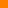Code (a software package for Matlab V7, 32-bit Linux, 64-bit Linux, 32-bit Windows), please cite us by . version 2 (new version) version 1Additional test images (a collection of several rectified images)

 left image right image disparity map (version 2)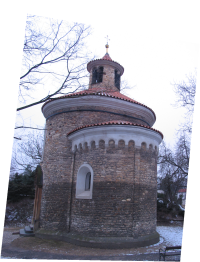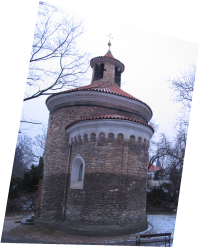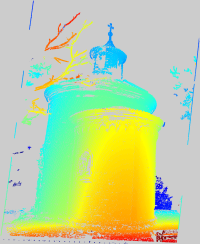image size = 1.8 Mpx CPU time = 8.9 sec.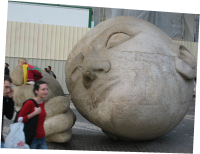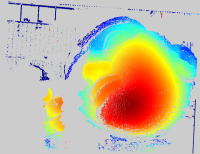image size = 1 Mpx CPU time = 7.0 sec.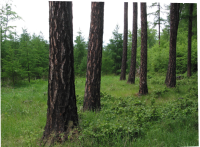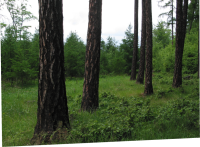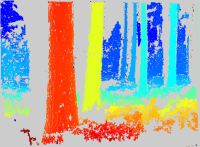image size = 0.5 Mpx CPU time = 2.1 sec.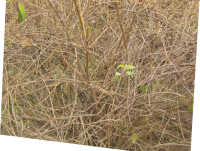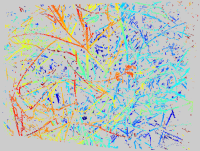image size = 5.3 Mpx CPU time = 31 sec.

The disparity maps ared coded in color (colder colors for larger depths, gray color for unassigned disparities), the CPU time  is measured on PC C2 2.4 GHz. Click on images to see them in a full resolution.

### Description

The algorithm we propose avoids visiting the entire disparity space by growing high similarity components in the disparity space. The final decision is performed by Confidently Stable Matching [2, 3] which selects among competing correspondence hypotheses. The algorithm is not a direct combination of unconstrained growth followed by a filtration step. Instead, the growing procedure is designed to fit the final matching algorithm in the sense the growing is stopped whenever the correspondence hypothesis cannot win the final matching competition.

Stereo algortihms are usually computationally intensive due to an inherent dimensionality of the problem. Assuming an epipolar rectification of two input images, the size of all possible pixel-to-pixel correspondence hypotheses, so called the disparity space, is n3, where the input image size is n2. For instance, for images 1000 x 1000 pixels, it is usually needed to exhaustively compute 109 of similarity statistics (correlation) which measure a quality of each correspondence hypothesis.

The proposed algorithm is fast. Matching almost 2 mega pixel image takes less than 10 seconds without limiting the disparity search range. This is mainly due to the fact that only a small fraction of disparity space (and hereby a small number of inter image similarity statistics) has to be computed. It is less than 1 per cent of the disparity space. This advantage becomes more apparent for larger images (above 1Mpx). Assuming the size of the images is n2, computational complexity is O(kn2) where k is a small constant which depends on the scene complexity determining the fraction of disparity space to be traversed. It always holds that k<<n. The complexity of the algorithm computing exhaustively the entire disparity space is O(n3).

The algorithm is described in paper .

Jan Cech,